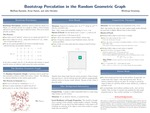## S.U.R.E Posters

Dr. Arran Hamm

#### College

College of Arts and Sciences

#### Department

Department of Mathematics

#### Description

Bootstrap percolation on a graph is a process which models the spread of an "infection" given an initially infected set of vertices of the graph. To state the problem more precisely, suppose G is a graph, k is a natural number, and I_0 is a set of initially infected vertices. Then for any discrete time t, we define I_t to be I_{t-1} along with any vertex outside of I_{t-1} which has at least k edges to vertices of I_{t-1}. This type of process may be used to model the spread of a disease by taking people as vertices, interactions between people as edges, and assuming a rate at which the infection spreads. That is, if the rate of spread is 10%, we would expect that if a person is in contact with 10 infected people, then the person will become infected. Other applications of this type of model involve the spread of rumors and the fault tolerance for distributed computing.

The random geometric graph is formed by fixing an r value and choosing n points from the unit square uniformly at random. We then join a pair of these points by an edge if their distance is less than r. This kind of random graph seems particularly relevant in the current socially distant world in which people attempt to only interact with others when necessary (i.e. distance-based edges roughly model this kind of relative isolation). Random geometric graphs have been well-studied. Bootstrap percolation on random geometric graphs has been examined although prior results in this direction cover limited regimes of the parameters. In our project, we extend previous work to study other ranges of values of the parameters. Along the way we use similar ideas to identify the threshold for connectivity in the random geometric graph which is a problem of independent interest.

10-5-2020

#### Disciplines

Mathematics

This project was supported by an Institutional Development Award from the National Institute of General Medical Sciences (2 P20 GM103499 20) of the National Institutes of Health.COinS